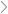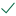Questions & AnswersFinancial AccountingThe company's current ratio ________ (Round to two decimal places)...

QuestionAnswered step-by-step

# The company's current ratio ________ (Round to two decimal places)...

The company's current ratio ________ (Round to two decimal places)

The company's times interest earned is ________ times. (Round to two decimal places)

The company's inventory turnover is _________ times (Round to two decimal places)

The company's total asset turnover is ___________ (Round to two decimal places)

The company's operating profit margin is __________ % (Round to one decimal place)

The company's operating return on assets is __________% (Round to one decimal place)

The company's debt ratio is ________ % (Round to one decimal place)

The company's average collection period is ________ days (Round to one decimal place)

The company's fixed asset turnover is ________  (Round to two decimal places)

The company's return on equity is _________ % (Round to one decimal place)

Image of grid:Solved by verified expert10,000 step-by-step explanations